Friday, 26 October 2018 15:46

## Modified Newtonian Dynamics in a Hypersphere. MOND(H) Featured

Abstract. This paper proposes a modification to Newton’s laws of motion and gravitation that would have negligible and undetectable effects at terrestrial scales, but at greater scales, it would have obvious effects that would create significant anomalies that we have already observed and that conventional theories have perhaps misinterpreted.

Newton devised his theories on the assumption that we inhabit a strictly Euclidian spacetime. Einstein showed that spacetime can have curvature. Conventional cosmology currently asserts that we inhabit an expanding universe with an overall curvature close to, or exactly equal to, zero. If however we entertain the possibility that the universe has a very small positive curvature then that small positive curvature could account for a number of apparent astronomical anomalies and remove the need to assume that it expands.

Modified Newtonian Dynamics in a Hypersphere. MOND(H)

If spacetime had a negative curvature and expanded continuously then we would expect relative velocities between objects to increase. Conversely, if spacetime had a positive curvature then we would expect the relative velocities between objects to decrease. In either case, the curvature of spacetime would appear as an acceleration.

Now if spacetime has a positive curvature, Newton’s first law of motion that ‘a body will continue in a state of rest or constant motion unless disturbed by an outside force’, undergoes a modification. The relative motion will decelerate over time.The deceleration A arises from the large-scale structure of the universe where M equals the mass of the universe and L equals the antipode length of a closed hyperspherical (3-ball) universe.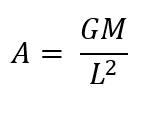This deceleration has already been observed in the Pioneer Anomaly where the residual deceleration of the spacecraft (after subtracting for thermal recoil) bottoms out at about 7 x 10^-10 m/s^-2

The deceleration A arising from a small positive spacetime curvature will also affect the behaviour of electromagnetic radiation passing across long distances. It cannot of course change the speed of light in a vacuum but it would change the wavelength (and frequency) as follows: -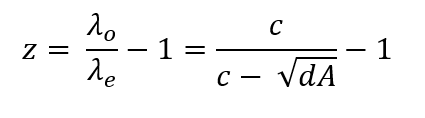To create a redshift.

A small positive spacetime curvature will also lead to a modification of Newton’s second law of motion that ‘force equals mass times acceleration’.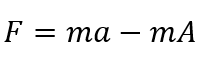This has immeasurably small effects on terrestrial mechanics, however consider its effects on Newton’s law of gravitation: -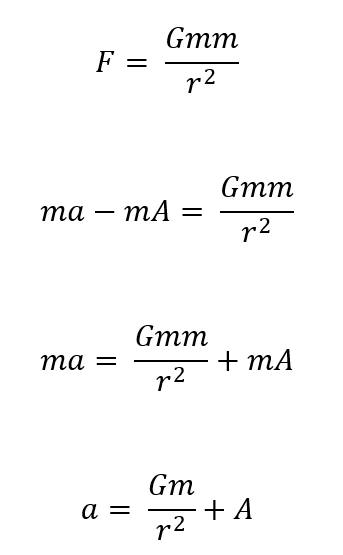(Positive spacetime curvature increases the acceleration due to gravity.)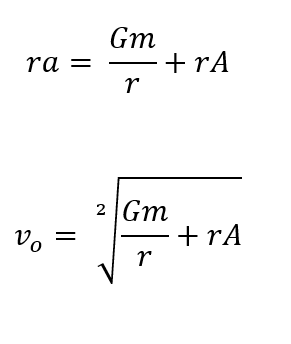Orbital velocity Vo will increase by a factor of rA. This will have negligible effects at planetary distances. However, at galactic distances it will have profound effects and flatten galactic rotation curves so that galaxies rotate like solid discs. This obviates the need for hypothetical dark matter or arbitrary adjustments to Newtonian dynamics at long distances.

Reference.

Hypersphere Cosmology 2. Author: Peter J Carroll. http://vixra.org/abs/1601.0026